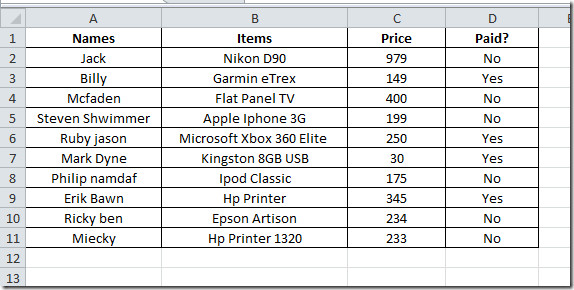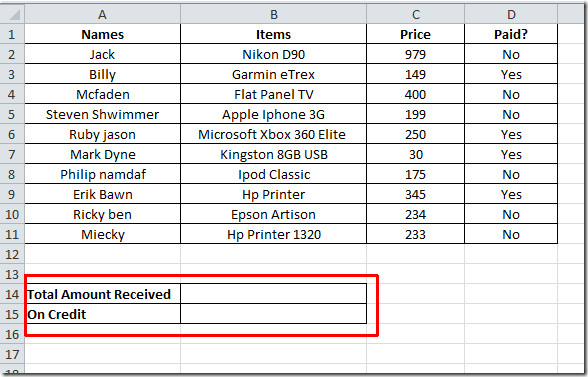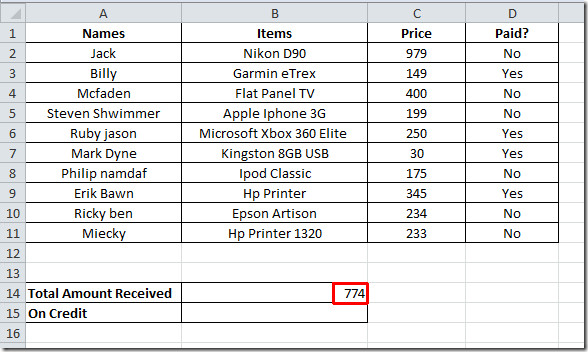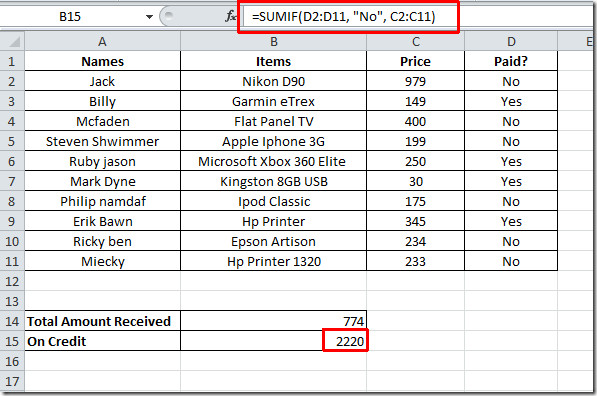# SUMIF Function In Excel 2010

In Excel 2010, by using SUMIF function we can calculate the sum of multiple data/entries based on desired condition. As name implies, it sums-up the data if the criteria is met. It takes three arguments in which we need to select range of data, criteria ,and sum_range to find out the desired result. This post elaborates a simple usage of SUMIF function.

Launch Excel 2010, open a datasheet on which you want to apply SUMIF conditional logic.

For Instance: We have a worksheet containing customer records with fields; Names, Items, Price and Paid?.Now we want to check the total amount paid by customers and total amount customers still owes. For that we need to add two new labels, Total Amount Received, and On Credit.Now we need to write a formula for Paid Amount, which yields sum of amount that has been paid by the customers.

Syntax:

SUMIF(range, criteria, sum_range)

Formula:

=SUMIF(D2:D11, “Yes”, C2:C11)

In formula, D2:D11 contains keywords Yes and No that show that whether customer paid the amount or still owes, C2:C11 contains Price of the corresponding product. By applying this formula it will show that sum of the amount paid by the customers by searching Yes in Paid? column and adding corresponding values in Price column. It will evaluate that 774\$ has been paid, as shown in the screenshot below.Now we will apply same formula to see how much amount customers still owes, for that we need a slight change in condition.The formula will search No in paid? column and sum up corresponding values in Price column.

=SUMIF(D2:D11, “No”, C2:C11)

It yields sum of amount owed by customers as shown in the screenshot.1.SCM says:

I have a spreadsheet where the “sumif” was copied to multiple ranges. The copied formula worked correctly for a week, then, in one cell, it was not working correctly. I copied from above, worked on it for 20 minutes trying to see what the problem was.
I then wrote the formula as originally, and it worked correctly.
this is 2nd time this has happened.
any ideas?

2.Walsh30167 says:

My value appears as 0 – Why is this, i have checked the format

3.LSK says:

How can I fix my Sumifs formula to NOT include highlighted entries?  Is this possible?

This site uses Akismet to reduce spam. Learn how your comment data is processed.